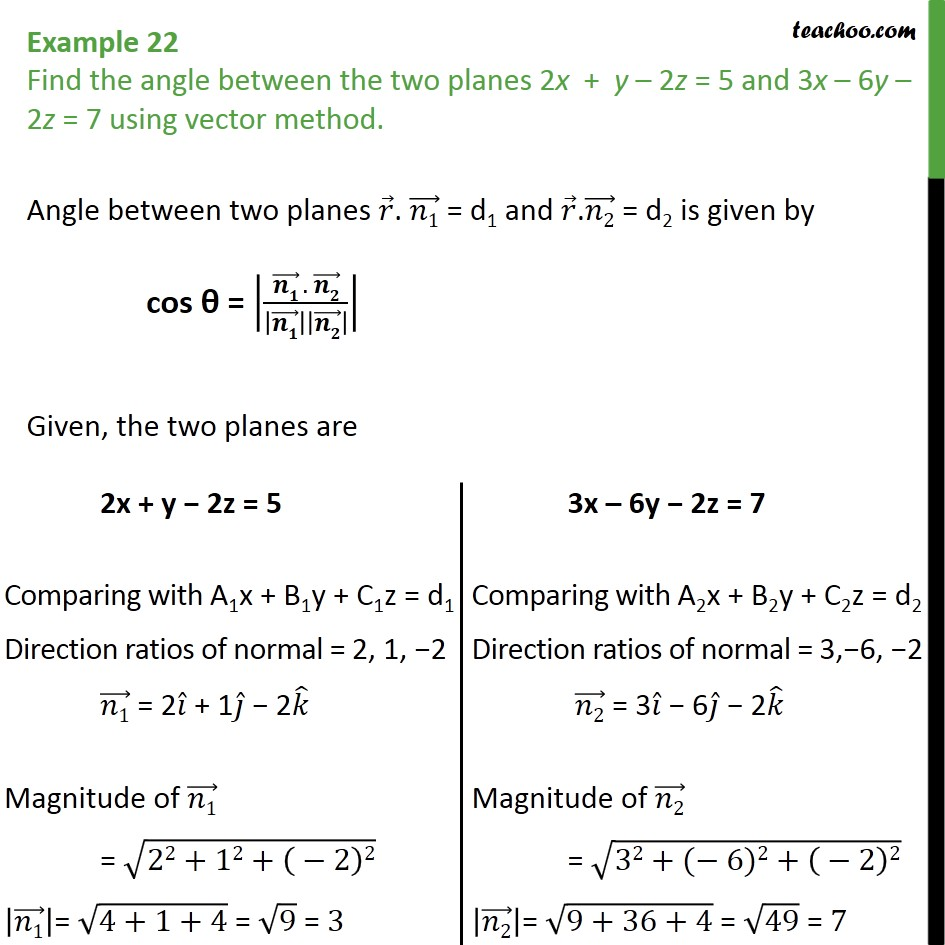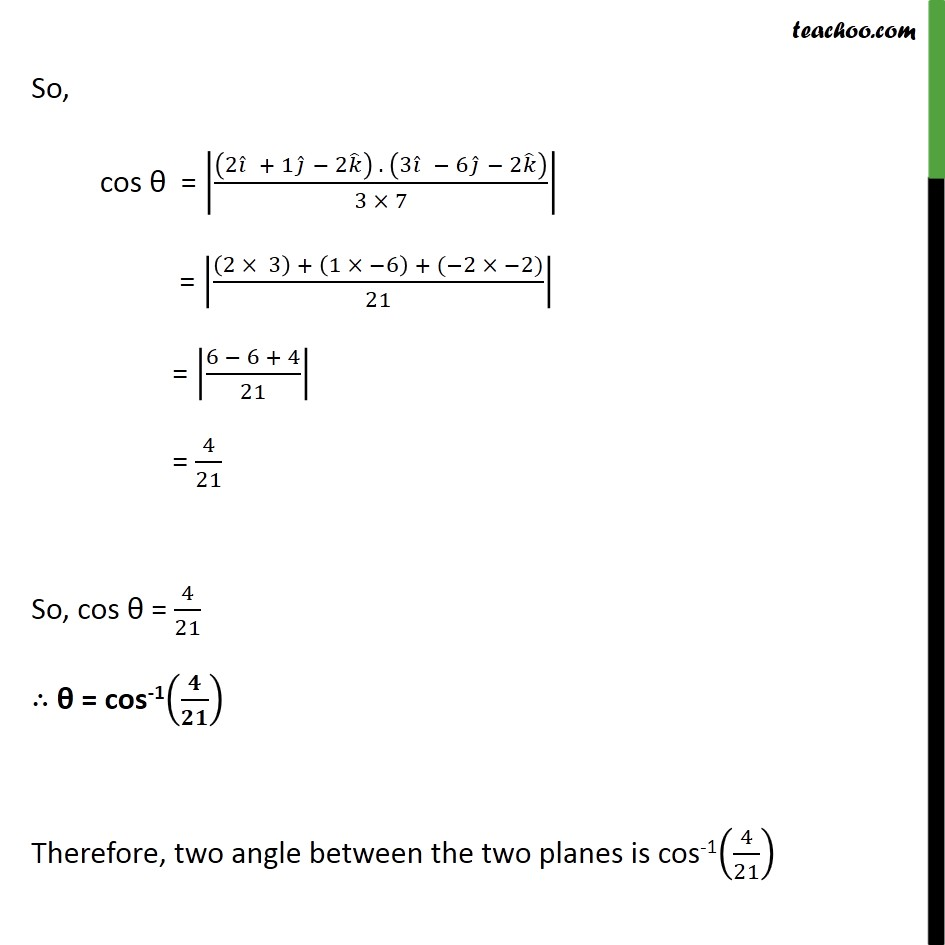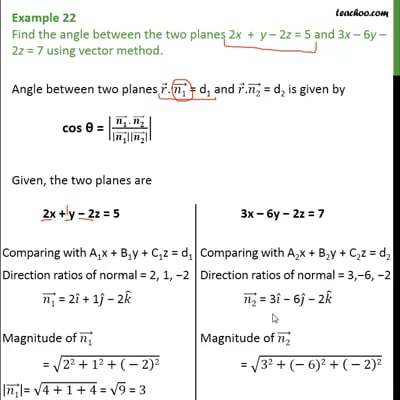Examples

Chapter 11 Class 12 Three Dimensional Geometry
Serial order wiseThis video is only available for Teachoo black users

Learn in your speed, with individual attention - Teachoo Maths 1-on-1 Class

### Transcript

Question 12 Find the angle between the two planes 2x + y 2z = 5 and 3x 6y 2z = 7 using vector method. Angle between two planes . ( 1) = d1 and .( 2) = d2 is given by cos = |(( ) . ( ) )/|( ) ||( ) | | Given, the two planes are 2x + y 2z = 5 Comparing with A1x + B1y + C1z = d1 Direction ratios of normal = 2, 1, 2 ( 1) = 2 + 1 2 Magnitude of ( 1) = (22+12+( 2)2) |( 1) |= (4+1+4) = 9 = 3 3x 6y 2z = 7 Comparing with A2x + B2y + C2z = d2 Direction ratios of normal = 3, 6, 2 ( 2) = 3 6 2 Magnitude of ( 2) = (32+( 6)2+( 2)2) |( 2) |= (9+36+4) = 49 = 7 So, cos = |((2 " " + 1 " " 2 ) . (3 " " 6 " " 2 ))/(3 7)| = |((2 3) + (1 6) + ( 2 2))/21| = |(6 6 + 4)/21| = 4/21 So, cos = 4/21 = cos-1( / ) Therefore, two angle between the two planes is cos-1(4/21)